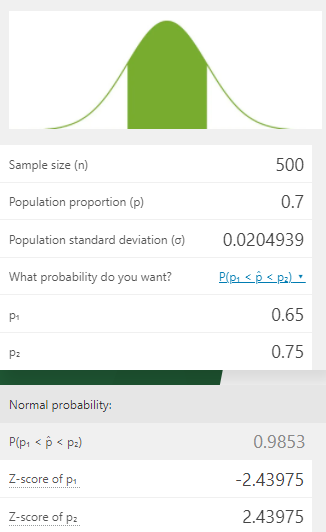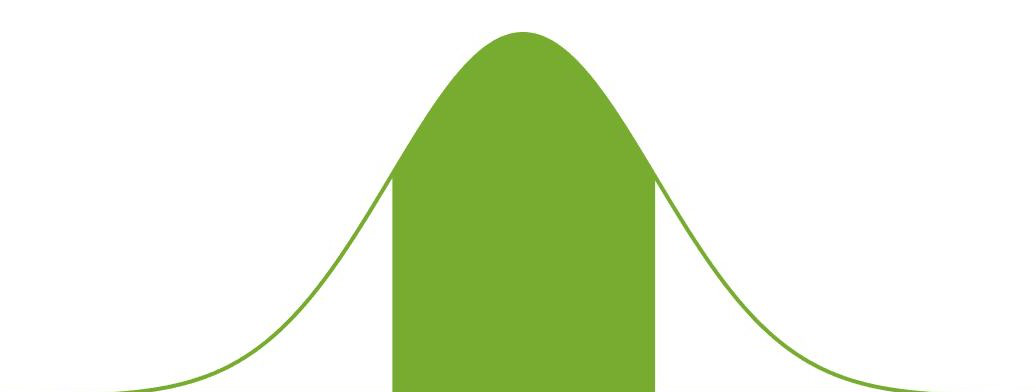# Sampling Distribution of the Sample Proportion Calculator

Created by Luis Hoyos
Reviewed by Steven Wooding
Last updated: Feb 02, 2023

This sampling distribution of the sample proportion calculator finds the probability that your sample proportion lies within a specific range: P(p₁ < p̂ < p₂), P(p₁ > p̂), or P(p₁ < p̂). It calculates the probability using the sample size (n), population proportion (p), and the specified proportions range (if you don't know the required n, use our sample size calculator).

Population proportion is vital in many scientific fields, as many research questions of interest involve this parameter. If you're interested in the opposite problem: finding a range of possible values of the population proportion given a confidence level, check our sampling error calculator. If, instead of calculating the sampling distribution of the sample proportion, you're interested in the mean, use our normal probability calculator for sampling distributions.

## What is the sampling distribution of the sample proportion?

To know what is the distribution of the sample proportion, we first have to talk about proportions. Proportions are one of the more predominant parameters in research. In plain words, they indicate the percentage of individuals with a specific characteristic. Examples are:

• The proportion of citizens who approve of how the president governs his country.
• Testing whether introducing physical activity diminishes cardiovascular disease incidence.
• The proportion of TVs of a particular model that fail after one year.

This parameter is straightforward to calculate. Divide the number of individuals who possess the characteristic by the total number of individuals, and you'll get it. As in the case of the mean, we usually use sample proportions to estimate population proportions.

If we took samples of and plotted each of the sample proportions encountered, we would end up with a sampling distribution, similar to what happens in the sampling distribution of the mean. The resulting distribution is called the sampling distribution of the sample proportion and is a graphical representation of the possible values of the population proportion.

Nevertheless, there are fundamental differences compared to the sampling distribution of the mean. The sample proportion is a discrete variable and not a continuous variable. Therefore, the sample proportion values are finite or countably infinite, unlike the sample mean case.

The sampling distribution of the sample proportion doesn't follow a normal distribution but a binomial distribution, which depends on the population proportion and the sample size. The good part is that, in most cases, we can approximate that discrete binomial distribution as a continuous normal distribution and use the widely known methods to find probabilities.

The approximation results in a normal distribution with mean $µ_{\hat p}$ and standard deviation $σ_p̂$, expressed in terms of the sample size $n$ and the population proportion $p$:

$\footnotesize µ_{\hat p} = p$
$\footnotesize σ_{\hat p} = \sqrt{\frac{p(1-p)}{n}}$

We use those values to find the z-scores and finally find the probability values, as we would usually do with a population mean:

$\footnotesize Z = \frac{p₀-µ_{\hat p}}{σ_p̂} = \frac{p₀-p}{σ_{\hat p}}$

🙋 In which cases does this approximation apply?
This approximation is inadequate for small sample sizes and p-values near 0 or 1. The following is a mathematical criterion for determining if the approximation holds well:

• np ≥ 15
• np(p-1) ≥ 15

The above equations imply there must be at least 15 successes and 15 failures for the approximation to be reasonably good.

The following image shows how proportions far from 0 and 1 and larger sample sizes approximate a normal distribution.

Let's look at an example of using these formulas and our sampling distribution of the sample proportion calculator.

## How to use this sampling distribution of the proportion calculator: an example

Suppose you know that 70% percent of the American population approves the management of the current president. If you conduct a pool and take a random sample of 500 people, what is the probability of finding at least 325 who support him?

1. Determine the proportion values.
• p = 70% = 0.7
• n = 500
• p₁ = 320/500 = 60% = 0.65
2. Input the $p$ and $n$ values in the calculator.
3. As we're interested in the values greater than p₁, select that you want "P(p̂ > p₁)."
4. Input p₁ = 0.65.
5. That's it. The answer is 0.992651, so the probability of finding at least this proportion of people who approve him is about 99.3%. In statistical notation: P(p̂ > 0.65) = 99.3%.

You can also check the results using the formulas shown before:

\footnotesize \begin{align*} σ_{\hat p} &= \sqrt{\frac{p(1-p)}{n}}\\\\ &= \sqrt{\frac{0.7(1-0.7)}{500}} = 0.0204939 \end{align*}
\footnotesize \begin{align*} Z &= \frac{p₁-p}{σ_{\hat p}}\\\\ &= \frac{0.65-0.7}{0.0204939} = -2.43975 \end{align*}
\footnotesize \begin{align*} P (p̂ > 0.65) &= P ( Z > −2.43975)\\ &= 0.992651 \end{align*}

Consider that $P ( Z > −2.43975)$ is not straightforward to calculate. To do it, you need a table to convert from z-score to P values, or you can use our p-value calculator (better and easier).

💡 If you want to calculate the probability of getting a $\hat p$ between two values, you have to select the option "P(p₁ < p̂ < p₂)" in the "What probability do you want" box. In the previous example, if you wanted to calculate the probability of finding a proportion of approvers between 0.65 and 0.75, your calculator should look this way:Do you have doubts about the calculations of the sampling distribution of the sample proportion? Maybe the following FAQs can help you.

## How to find the sample proportion?

Follow these steps to find the sample proportion:

1. Determine the number of successes in your sample.
3. Divide the number of successes by the sample size. This result represents the fraction or percentage of successes in your sample. That's how you find the sample proportion.

## How to find the standard deviation of sample proportion?

The process of finding the standard deviation of the sample proportion depends on the available information:

• If you know the population proportion (p) and the sample size (n), input those values in the sample proportion standard deviation formula: √[p (p - 1)/n].
• If you only know the sample proportion (p̂ ) and the sample size (n), input those values in the standard error of the sample proportion formula: √[p̂ (p̂ - 1)/n], which is an estimate of the sample proportion standard deviation.

## FAQ

### What is the difference between population proportion and sample proportion?

• The population proportion (p) is the number of successes (X) of the entire population divided by the population size (N): p = X/N.
• The sample proportion (p̂ ) is the number of successes found in the sample (x) divided by the sample size (n): p̂ = x/n. That's how you find the sample proportion.

### What is the probability of getting a sample proportion higher than the population proportion?

The probability of getting a sample proportion higher than the population proportion is 50% as long as the sampling distribution of the proportion is symmetrical. The larger the sample size or the farthest the proportion value is from 0 or 1, the more symmetric the distribution.

Luis HoyosSample size (n)
Population proportion (p)
Population standard deviation (σ)
What probability do you want?
P(p₁ < p̂ < p₂)
p₁
p₂
Normal probability:
P(p₁ < p̂ < p₂)
Z-score of p₁
Z-score of p₂
People also viewed…

### Chi-square

Determine the goodness of fit of your data with the chi-square calculator.

### Korean age

If you're wondering what would your age be from a Korean perspective, use this Korean age calculator to find out.

### Plant spacing

Planning out your garden? Try the plant spacing calculator.

### Quartic regression

A set of x-y data is fitted with a 4th-order polynomial model using the quartic regression calculator.